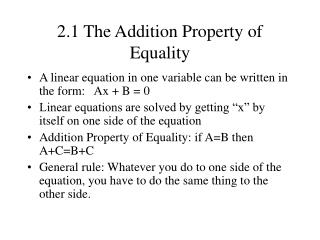DownloadDownload Presentation2.1 The Addition Property of Equality

# 2.1 The Addition Property of Equality

Download Presentation## 2.1 The Addition Property of Equality

- - - - - - - - - - - - - - - - - - - - - - - - - - - E N D - - - - - - - - - - - - - - - - - - - - - - - - - - -
##### Presentation Transcript

1. 2.1 The Addition Property of Equality • A linear equation in one variable can be written in the form: Ax + B = 0 • Linear equations are solved by getting “x” by itself on one side of the equation • Addition Property of Equality: if A=B then A+C=B+C • General rule: Whatever you do to one side of the equation, you have to do the same thing to the other side.

2. 2.1 The Addition Property of Equality • Example of Addition Property:x – 5 = 12 (add 5 to both sides)x = 17 • Example using subtraction:

3. 2.2 The Multiplication Property of Equality • Multiplication Property of Equality: if A=B and C is non-zero, then AC=BC (both equations have the same solution set) • Since division is the same as multiplying by the reciprocal, you can also divide each side by a number. • General rule: Whatever you do to one side of the equation, you have to do the same thing to the other side.

4. 2.2 The Multiplication Property of Equality • Example of Multiplication Property: • Example using division5x = 60 (divide both sides by 5)x = 12

5. 2.3 More on Solving Linear Equations: Terms - Review • As with expressions, a mathematical equation is split up into terms by the +/-/= sign: • Remember, if the +/- sign is in parenthesis, it doesn’t count:

6. 2.3 More on Solving Linear Equations: Multiplying Both Sides by a Number • Multiply each term by the number (using the distributive property). • Within each term, multiply only one factor.Notice that the y+1 does not become 4y+4

7. 2.3 More on Solving Linear Equations: Clearing Fractions • Multiply both sides by the Least Common Denominator (in this case the LCD = 4):

8. 2.3 More on Solving Linear Equations: Clearing Decimals • Multiply both sides by the smallest power of 10 that gets rid of all the decimals

9. 2.3 More on Solving Linear Equations: Why Clear Fractions? • It makes the calculation simpler:

10. 2.3 More on Solving Linear Equations • 1 – Multiply on both sides to get rid of fractions • 2 – Use the distributive property to remove parenthesis • 3 – Combine like terms • 4 – Put variables on one side, numbers on the other by adding/subtracting on both sides • 5 – Get “x” by itself on one side by multiplying both sides • 6 – Check your answers

11. 2.3 More on Solving Linear Equations • Example:

12. 2.4 An Introduction to Applications for Linear Equations • 1 – Decide what you are asked to find • 2 – Write down any other pertinent information (use other variables, draw figures or diagrams ) • 3 – Translate the problem into an equation. • 4 – Solve the equation. • 5 – Answer the question posed. • 6 – Check the solution.

13. 2.4 An Introduction to Applications for Linear Equations • Find the measure of an angle whose complement is 10 larger. • x is the degree measure of the angle. • 90 – x is the degree measure of its complement • 90 – x = 10 + x • Subtract 10: 80 – x = xAdd x: 80 = 2xDivide by 2: x = 40 • The measure of the angle is 40  • Check: 90 – 40 = 10 + 40

14. A = lw I = prt P = a + b + c d = rt V = LWH C = 2r Area of rectangle Interest Perimeter of triangle Distance formula Volume – rectangular solid Circumference of circle 2.5 Formulas - examples

15. Example: d=rt; (d = 252, r = 45)then 252 = 45tdivide both sides by 45: 2.5 Formulas

16. 2.6 Ratios and Proportions • Ratio – quotient of two quantities with the same unitsExamples: a to b, a:b, or Note: percents are ratios where the second number is always 100:

17. 2.6 Ratios and Proportions • Proportion – statement that two ratios are equalExamples: Cross multiplication:if then

18. 2.6 Ratios and Proportions • Solve for x: Cross multiplication:so x = 63

19. 2.7 More about Problem Solving • Percents are ratios where the second number is always 100: Example: If 70% of the marbles in a bag containing 40 marbles are red, how many of the marbles are red?:#red marbles =

20. 2.7 More about Problem Solving • How many gallons of a 12% indicator solution must be mixed with a 20% indicator solution to get 10 gallons of a 14% solution? Let x= #gallons of 12% solution,then 10-x= #gallons of 20% solution :

21. 2.8 Solving Linear Inequalities • < means “is less than” •  means “is less than or equal to” • > means “is greater than” •  means “is greater than or equal to”note: the symbol always points to the smaller number

22. 2.8 Solving Linear Inequalities • A linear inequality in one variable can be written in the form: ax < b (a0) • Addition property of inequality:if a < b then a + c < b + c

23. 2.8 Solving Linear Inequalities • Multiplication property of inequality: • If c > 0 thena < b and ac < bc are equivalent • If c < 0 thena < b and ac > bc are equivalentnote: the sign of the inequality is reversed when multiplying both sides by a negative number

24. 2.8 Solving Linear Inequalities • Example: -9